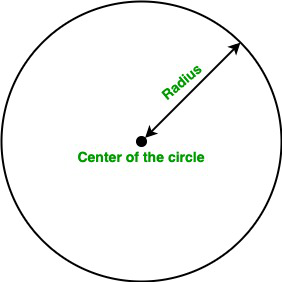GeeksforGeeks App
Open AppBrowser
Continue

# Java Program to Find the Area of a Circle Given the Radius

A circle is a simple shape consisting of all the points in the plane that are equidistant from a point known as the center of the circle. In this article, we will learn how to find the area of the circle.

Terminology:

• Area: A quantity that represents the extent of a 2-dimensional figure or shape in the plane is known as an area.
• Radius: The line segment from the center to any point of the circle is known as radius.
• Diameter: The line segment whose endpoints lie on the circle and passed through the center is known as the diameter of the circle. It is also known as the largest distance between any two points on the circle.

Area of the Circle

The area of the circle is the product of the square of the radius of the circle and the value of PI. We can simply calculate the area of the circle using the following formula:

• Using the radius of the circle:Formula:

Area of the circle:  A = π * r2

Here, r is the radius of the circle.

Note: The value of PI in java is 3.141592653589793.

Below is the implementation of the above approach:

Example:

## Java

 `// Java program to find``// the area of the circle` `import` `java.io.*;` `class` `GFG {` `    ``static` `final` `double` `PI = Math.PI;` `    ``// Function to calculate the``    ``// area of the circle``    ``static` `double` `Area(``double` `r) { ``return` `PI * r * r; }` `    ``// Driver code``    ``public` `static` `void` `main(String[] args)``    ``{` `        ``// Radius``        ``double` `r = ``5``;` `        ``// Calling Area function``        ``System.out.println(``"Area of the circle is :"``                           ``+ Area(r));``    ``}``}`

Output

`Area of the circle is :78.53981633974483`

Time complexity: O(1) since performing constant operations

Auxiliary Space: O(1)

My Personal Notes arrow_drop_up